Covariance analysis

A collection of methods in mathematical statistics relating to the analysis of models of the dependence of the mean value of some random variableon a set of non-quantitative factorsand simultaneously on a set of quantitative factors. The variablesare called the concomitant variables relative to; the factorsdefine a set of conditions of a qualitative nature under which the observations onandare obtained, and are described by so-called indicator variables; among the concomitant and indicator variables can be both random and non-random ones (controlled in the experiment); if the random variableis a vector, then one talks about multivariate analysis of covariance.
The basic theoretic and applied problems in the analysis of covariance relate to linear models. For example, if the scheme under analysis consists ofobservations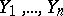withconcomitant variables andpossible types of experimental conditions, then the linear model of the corresponding analysis of covariance is defined by the equations(*)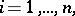where the indicator variables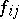are equal to 1 if the-th experimental condition prevails for the observation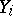and 0 otherwise; the coefficientsmeasure the influence of the-th condition;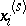is the value of the concomitant variable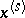for whichis obtained;;; the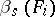are the values of the corresponding regression coefficients ofonwhich, in general, depend on the concrete combination of the conditions of the experiment, that is, on the vector; the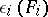are random errors having zero mean values. The main content of the analysis of covariance is the construction of statistical estimators for the unknown parameters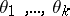;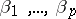, and of statistical criteria for testing various hypotheses about the values of these parameters.
If in the model (*) one postulates a priori that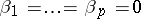, then a dispersion analysis model is obtained; if in (*) one excludes the influence of the non-quantitative factors (by setting), then a regression analysis model is obtained. The terminology "analysis of covariance" refers to the fact that in its calculations one makes use of the decomposition of the covariance ofandin precisely the same way as the decomposition of the sum of squares of the deviations ofis used in dispersion analysis.